# Sample Problem Force And Motion

Sample Problem Force And Motion

Let us return to John Massis and the railroad cars, and assume that Massis pulled (with his teeth) on his end of the rope with a constant force that was 2.5 times his body weight. at an angle (J of 30° from the horizontal. His mass m was 80 kg. The weight W of the cars was 700 km. and he moved them 1.0 m along the rails. Assume that the rolling wheels encountered no retarding force from the rails. What was the speed of the cars at the end of the pull?

SOLUTION: A Key Idea here is that, from Newton’s second law, the constant horizontal force on the cars from Massis causes a constant horizontal acceleration of the cars. Because the acceleration is constant and the motion is one-dimensional, we can use the equations  to find the velocity . at the end of the pulling distance d = 1.0 m. We need an equation that contains.

where M is the mass of the cars. The only force on the cars along the v axis is the horizontal component T cos 8 of the tension force f on the cars from the rope pulled by Massis. Thus,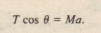We still need M in order to evaluate  for a, To find M. we again use . but now with til weight W of the cars .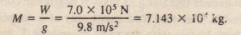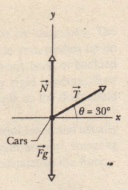Free bodydiagram for the passenger carspulled by Massis. The vectors are notdrawn to scale; the force f on the carsfrom the rope is much smaller than thenormal force N on the cars from therails and the gravitational force F. onthe cars.

By rearranging and substituting for T, M. and 8, we get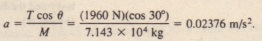Substituting this value and the other known values into now gives us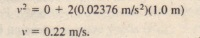Massis would have done better if the rope had been attached higher non the car, so that it was horizontal. Can you see why?